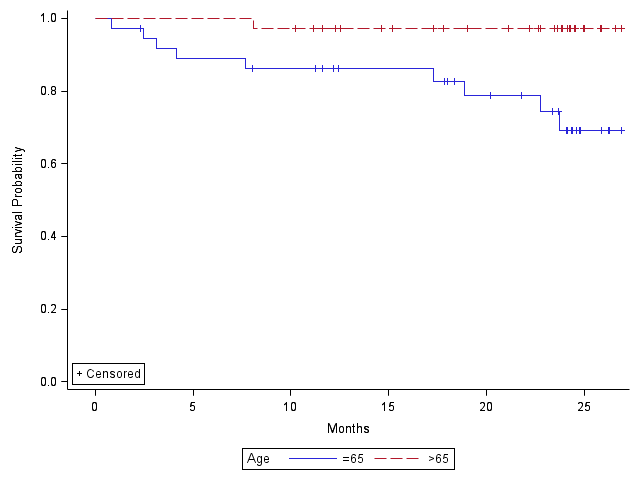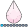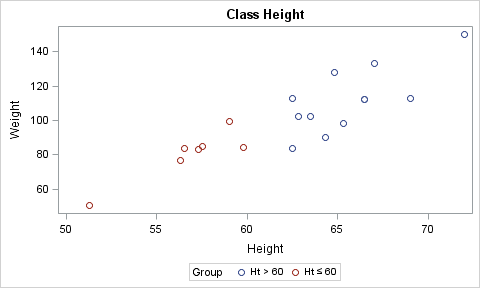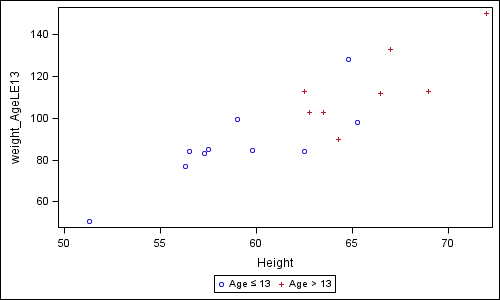## Why legend cannot show symbol ≤ in a kaplan-Meier curve?

Dear All,

Here is my syntax:

ods listing sge = on ;
proc lifetest data = primary2 plot=(s) graphics;
time month*status(0);
strata agecat;
format agecat agecat.;
title'survival analysis based on age';
run;

Here is my format:
value agecat
0= '≤65'
1= '>65';

Graph is attached.

Is there a way to show symbol ≤ in the graph?1 ACCEPTED SOLUTION

Accepted Solutionsdjrisks
Barite | Level 11

## Re: Why legend cannot show symbol ≤ in a kaplan-Meier curve?

Hello,

One way to do this, is to use the Unicode, i.e. "^{unicode '2264'x} 65"

Firstly though, we need to output a dataset and this can be done using the OUTSURV statement in Proc Lifetest, and then we can use GTL to produce the KM figure.

You can adapt the code below to display the sign you want.

data BMT;
set sashelp.BMT;
if group = "ALL" then groupn = 1;
else if group = "AML-Low Risk" then groupn = 2;
else if group = "AML-High Risk" then groupn = 3;
run;

proc lifetest data=BMT plots=survival(atrisk=0 to 2500 by 500) outsurv = outsurv;
time T * Status(0);
run;

ods escapechar  = "^";

proc template;
define statgraph kaplan;
begingraph;
layout overlay / yaxisopts = (label = "Survial Probability") xaxisopts = (label = "Months");

stepplot y = eval(ifn(stratum = 1, survival, .)) x = eval(ifn(stratum = 1, t, .)) / name = "leg" legendlabel = "^{unicode '2264'x} 65" lineattrs = GRAPHDATA1;
scatterplot y = eval(ifn(stratum = 1 and _CENSOR_ = 1, survival, .)) x = eval(ifn(stratum = 1 and _CENSOR_ = 1, t, .)) / markerattrs = (color = GRAPHDATA1:color symbol = plus);

stepplot y = eval(ifn(stratum = 2, survival, .)) x = eval(ifn(stratum = 2, t, .)) / name = "leg2" legendlabel = "> 65" lineattrs = GRAPHDATA2;
scatterplot y = eval(ifn(stratum = 2 and _CENSOR_ = 1, survival, .)) x = eval(ifn(stratum = 2 and _CENSOR_ = 1, t, .)) / markerattrs = (color = GRAPHDATA2:color symbol = plus);

discretelegend "leg" "leg2";

endlayout;
endgraph;
end;
run;

proc sgrender data = outsurv template = kaplan;
run;

13 REPLIES 13

## Re: Why legend cannot show symbol ≤ in a kaplan-Meier curve?

The issue is likely caused by the character set you're using (I assume it's single byte). Below code shows you what's available to you in single byte.

data _null_;

put "Session encoding is: &SYSENCODING";

do i=0 to 255;

char=BYTE (i);

put i= @10 char;

end;

stop;

run;

It's possible to use more characters but then you need to switch over to MBCS (eg. UTF-8): SAS(R) 9.4 National Language Support (NLS): Reference Guide, Third Edition

## Re: Why legend cannot show symbol ≤ in a kaplan-Meier curve?

Hi Patrick,

Run your code, I cannot find symbol ≤ in my SAS 9.4.   Should I use encoding=UTF-8 for ods statement to solve my problem? Can you teach me how to do it?

Thanks

## Re: Why legend cannot show symbol ≤ in a kaplan-Meier curve?

It's not only about printing but already about passing a multi-byte value to a format and then store such a value as part of the format.

You could make your life much easier by using single byte like below:

proc format;

value agecat

0= '<= 65'

1= '> 65'

;

run;djrisks
Barite | Level 11

## Re: Why legend cannot show symbol ≤ in a kaplan-Meier curve?

Hello,

One way to do this, is to use the Unicode, i.e. "^{unicode '2264'x} 65"

Firstly though, we need to output a dataset and this can be done using the OUTSURV statement in Proc Lifetest, and then we can use GTL to produce the KM figure.

You can adapt the code below to display the sign you want.

data BMT;
set sashelp.BMT;
if group = "ALL" then groupn = 1;
else if group = "AML-Low Risk" then groupn = 2;
else if group = "AML-High Risk" then groupn = 3;
run;

proc lifetest data=BMT plots=survival(atrisk=0 to 2500 by 500) outsurv = outsurv;
time T * Status(0);
run;

ods escapechar  = "^";

proc template;
define statgraph kaplan;
begingraph;
layout overlay / yaxisopts = (label = "Survial Probability") xaxisopts = (label = "Months");

stepplot y = eval(ifn(stratum = 1, survival, .)) x = eval(ifn(stratum = 1, t, .)) / name = "leg" legendlabel = "^{unicode '2264'x} 65" lineattrs = GRAPHDATA1;
scatterplot y = eval(ifn(stratum = 1 and _CENSOR_ = 1, survival, .)) x = eval(ifn(stratum = 1 and _CENSOR_ = 1, t, .)) / markerattrs = (color = GRAPHDATA1:color symbol = plus);

stepplot y = eval(ifn(stratum = 2, survival, .)) x = eval(ifn(stratum = 2, t, .)) / name = "leg2" legendlabel = "> 65" lineattrs = GRAPHDATA2;
scatterplot y = eval(ifn(stratum = 2 and _CENSOR_ = 1, survival, .)) x = eval(ifn(stratum = 2 and _CENSOR_ = 1, t, .)) / markerattrs = (color = GRAPHDATA2:color symbol = plus);

discretelegend "leg" "leg2";

endlayout;
endgraph;
end;
run;

proc sgrender data = outsurv template = kaplan;
run;

## Re: Why legend cannot show symbol ≤ in a kaplan-Meier curve?

Hi Patrick and djrisks, Thanks your help.

## Re: Why legend cannot show symbol ≤ in a kaplan-Meier curve?

If it works, please mark question as answeredJay54
Meteorite | Level 14

## Re: Why legend cannot show symbol ≤ in a kaplan-Meier curve?

FYI, something to look forward to in SAS 9.4M3.  See legend in this graph.

Unicode strings in User Defined Formats will be supported in both GTL and SG across the board.  You can use this to get such character strings into the legend, or the axis tick values or data labels.  Note:  Unicode strings can be included in the axis labels by using the LABEL option on the axis since SAS9.2.

{SUP} and {SUB} still cannot be supported. But most numeric sub and super scripts can be found in Unicode fonts.## Re: Why legend cannot show symbol ≤ in a kaplan-Meier curve?

Hi Sanjay,

I got my SAS 9.4 TS level1 M1last September. Is the SAS 9.4 M3 available soon? I used <= for now which I am not sure the journal will like it.

Thanks,

Xinjiandjrisks
Barite | Level 11

## Re: Why legend cannot show symbol ≤ in a kaplan-Meier curve?

Hi Xinjian.

Just letting you know that you can also obtain the correct "≤" using the example that I showed. If you have any questions about that example, please let me know.

Thanks.

## Re: Why legend cannot show symbol ≤ in a kaplan-Meier curve?

hi djrisks,

I got it.

Thanksdjrisks
Barite | Level 11

## Re: Why legend cannot show symbol ≤ in a kaplan-Meier curve?

You're welcome xinjian!Jay54
Meteorite | Level 14

## Re: Why legend cannot show symbol ≤ in a kaplan-Meier curve?

The issue is of supporting the Unicode values when they come from data, like group values.  Unicode values are supported when they are provided in a SG or GTL option, like LABEL or LEGENDLABEL.  But not in the data itself as yet.  With SAS 9.4M3 (release this summer) will allow this with Unicode in UDF.

If this is really necessary, and you are willing to write a bit more code, you can get what you want with some customization.  See my program and graph below.  I have essentially created multi-column data for weights for kids <= 13 and over 13 (instead of a group variable).  Now, I plot two scatter plots with each of the data columns against "Height".  Now, for each scatter plot, I assign the LEGENDLABEL I want including the Unicode value.  Note:  This will work with SAS 9.2 and above.

With Survival data, you will have to run proc Lifetest, and get back the survival data for plotting.  Then restructure the data from GROUP case to Multi-Column. Now, use your own SGPLOT program with Series overlays with LEGENDLABELS instead of a Grouped series plot.  See various examples in Graphically Speaking for Survival Plots using SGPLOT.data class;

keep name height weight_AgeLE13 weight_AgeGT13;

set sashelp.class;

if age le 13 then weight_AgeLE13=weight;

else weight_AgeGT13=weight;

run;

proc sgplot data=class;

scatter x=height y=weight_AgeLE13 / legendlabel="Age ~{Unicode  '2264'x} 13";

scatter x=height y=weight_AgeGT13 / legendlabel="Age > 13";

run;djrisks
Barite | Level 11

## Re: Why legend cannot show symbol ≤ in a kaplan-Meier curve?

That's great to hear Sanjay. I'm looking forward to using that.

Discussion stats
• 13 replies
• 2443 views
• 12 likes
• 5 in conversation